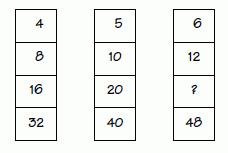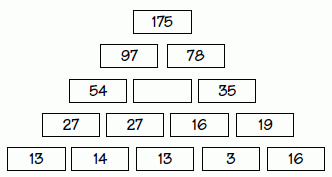## Numbers Puzzles

5. Can you determine the missing number in the box? The same rule of logic applies to all three boxes?Answer
 The missing number is 24. The rule is to double the first number in each rectangle and place that number in the next box below it.
6. Below is a pyramid of numbers where the number on each brick is the sum of two bricks below it. The numbers given will help you fill in the entire pyramid.Answer
 43